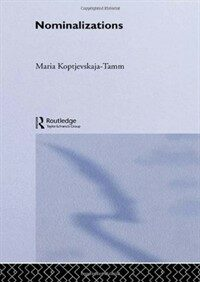> Detail View

# Detail View## Nominalizations (Loan 2 times)

Material type
단행본
Personal Author
Koptjevskaja-Tamm, Maria.
Title Statement
Nominalizations / Maria Koptjevskaja-Tamm.
Publication, Distribution, etc
London ; :   Routledge,   1993.
Physical Medium
xviii, 333 p. ; 22 cm.
Series Statement
Theoretical linguistics
ISBN
0415060206
Bibliography, Etc. Note
Includes bibliographical references (p. -323) and indexes.
비통제주제어
Grammar,,
 000 00817camuuu200289 a 4500 001 000000476232 003 OCoLC 005 19970418141339.0 008 920225s1993 enk b 001 0 eng 010 ▼a 92007229 015 ▼a GB93-14736 019 ▼a 28708023 020 ▼a 0415060206 040 ▼a DLC ▼c DLC ▼d UKM 049 1 ▼a ACCL ▼l 111084514 050 0 0 ▼a P271 ▼b .K67 1993 082 0 0 ▼a 415 ▼2 20 090 ▼a 415 ▼b K83n 100 2 ▼a Koptjevskaja-Tamm, Maria. 245 1 0 ▼a Nominalizations / ▼c Maria Koptjevskaja-Tamm. 260 ▼a London ; : ▼b Routledge, ▼c 1993. 300 ▼a xviii, 333 p. ; ▼c 22 cm. 490 1 ▼a Theoretical linguistics 504 ▼a Includes bibliographical references (p. -323) and indexes. 653 0 ▼a Grammar 830 0 ▼a Theoretical linguistics (Routledge (Firm))

### Holdings Information

No. Location Call Number Accession No. Availability Due Date Make a Reservation Service
No. 1 Location Call Number 415 K83n Accession No. 452094328 Availability Available Due Date Make a Reservation Service

### Contents information

```
CONTENTS
Acknowledgements = xiii
Abbreviations = xv
Part 1 Theoretical background = 1
1. Preliminaries: the aim of this book = 3
1.1. Goals of the study = 3
1.2. Stating the problem = 5
1.3. Illustrating the problem: internal syntax of Russian ANCs = 8
1.4. The problem of arguments = 9
1.5. Semantics of ANCs = 15
2. ANCs as non-finite noun clauses = 22
2.0. Introduction = 22
2.1. Subordination, 'balancing' and 'deranking' = 22
2.2. Types of noun clauses = 24
2.3. Language specific variations in the semantic range of ANCs, some examples = 45
2.4. Lexical nominalization vs. clausal nominalization = 49
3. Noun phrases vs. finite clauses in different languages = 53
3.0. Introduction = 53
3.1. Noun phrases vs. finite clauses - a universal opposition? = 54
3.2. Discussion of the basis for a classification of ANCs across languages = 58
3.3. The typology of ANCs = 60
3.4. Isomorphism between NPs and finite clauses : formulating the problem = 65
3.5. Affinities between noun phrases and finite clauses: more examples = 69
Part 2 The data = 77
4. The data base = 79
4.0. Introduction = 79
4.1. Obtaining data = 79
4.2. Characteristics of the sample = 82
5. Type 1: 'Sentential' = 90
5.0. Introduction = 90
5.1. 'Ideal' representatives: Korean and Archi = 90
5.2. Problems in defining the pattern = 94
5.3. Head-marking in the SENT type = 98
5.4. Further characteristics of the SENT type = 102
5.5. Intra-lexemic syntactic variation = 103
5.6. Summary = 108
6. Type 2: 'Possessive-Accusative' = 110
6.0. Introduction = 110
6.1. 'Ideal' representatives: Selkup and West Greenlandic = 110
6.2. Problems in defining the pattern = 116
6.3. Head-marking in the POSS-ACC type = 120
6.4. Further characteristics of the POSS-ACC type = 122
6.5. Intra-lexemic syntactic variation = 126
7. Type 3: 'Ergative-Possessive' = 129
7.0. Introduction = 129
7.1. Languages with agentive passives = 130
7.2. Pure OBL-POSS nominalizations vs. passives:possible connections = 137
7.3. Languages without agentive passives = 156
7.4. Head-marking in the ERG-POSS type = 159
7.5. Further characteristics of the pure ERG-POSS type = 159
7.6. Intra-lexemic syntactic variation = 161
7.7. Summary = 161
8. Type 4: 'Nominal' = 165
8.0. Introduction = 165
8.1. The DBL-POSS subtype = 165
8.2. The POSS-ADN subtype = 170
8.3. Summary = 180
9. Less frequent types = 181
9.0. Introduction = 181
9.1. Type 5: 'Mixed' = 181
9.2. Type 6: 'Incorporating' = 184
9.3. Type 7: 'Relative' = 191
9.4. Type 8: 'Argument-Reducing' = 194
10. Splits and variation = 197
10.0. Introduction = 197
10.1. Lexical, semantic and functional splits = 197
10.2. Referentiality/animacy split = 201
10.3. Ambiguities = 203
10.4. Alienable vs. inalienable possession and ANCs = 210
10.5. Pattern shifts and intra-lexemic variation = 214
Part 3 Discussion = 215
11. Explanation of type-membership = 221
11.0. Introduction = 221
11.1. Genetic and areal distribution of the nominalization types = 222
11.2. Complement systems = 223
11.3. Word order = 229
11.4. The structure of non-derived NPs = 234
11.6. Nominativity vs. ergativity = 241
11.7. Morphological type = 245
12. Explanation of the attested types = 247
12.0. Introduction = 247
12.1. Finite clauses vs. NPs vs. ANCs: direct analogy or modelling? = 248
12.2. Sentence-like vs. NP-like properties of ANCs = 254
12.3. ANCs as products of competing motivations = 257
12.4. Inflection vs. derivation and ANCs = 263
12.5. Why an action nominal construction? = 266
Summary = 271
Appendix A: Alphabetical language index = 273
Appendix B: ANC types with examples = 280
Notes = 300
Bibliography = 307
Author index = 324
Language index = 327
Subject index = 330

```

### New Arrivals Books in Related Fields

#### Grammaticalization / 1st ed

Narrog, Heiko (2021)